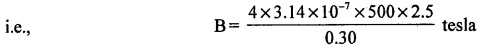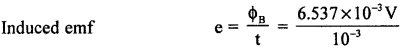# 2nd PUC Physics Question Bank Chapter 6 Electro Magnetic Induction

Students can Download 2nd PUC Physics Chapter 6 Electro Magnetic Induction Questions and Answers, Notes Pdf, 2nd PUC Physics Question Bank with Answers helps you to revise the complete Karnataka State Board Syllabus and to clear all their doubts, score well in final exams.

## Karnataka 2nd PUC Physics Question Bank Chapter 6 Electro Magnetic Induction

### 2nd PUC Physics Electro Magnetic Induction One Marks Questions and Answers

Question 1.
What is electromagnetic induction?
The emf and hence current induced in a circuit due to the change in magnetic flux linked with it is called electromagnetic induction.

Question 2.
When is emf induced in a circuit?
The emf is induced in a circuit whenever there is a change in the magnetic flux passing through it.

Question 3.
When is current induced in a circuit?
A current is induced in a circuit whenever there is a change in the magnetic flux linked with the closed circuit.Question 4.
State Lenz’s law.
Lenz’s law states that, the induced current is always in such a direction as to oppose the cause which produces it.

Question 5.
State Faraday’s law of electromagnetic induction. (July 2014)
It states that whenever there is a change in the magnetic flux linked with a circuit an emf is induced in it and the magnitude of the induced emf is directly proportional to the rate of change of magnetic flux.

Question 6.
Write Neumann’s relation.
e = $$-\frac{d \phi}{d t}$$

Question 7.
What is self induction?
The phenomenon in which an emf induced in a coil due to the change of current through it is called self induction. The S.I. unit of self inductance is henry (H).

Question 8.
Define self inductance.
Inductance of a coil is numerically equal to the emf induced in it, when the rate of change of current through it is unity.

Question 9.
Define henry.
The self inductance of a coil is said to be one henry, if one volt of emf is induced in the coil when current through it changes at the rate of one ampere per second.

Question 10.
What is mutual induction?
The phenomenon in which a change of current in one coil induces an emf in another coil placed in its proximity is called mutual inductance.Question 11.
What will be the induced current in a circuit placed in a constant magnetic field?
Zero.

Question 12.
Mention the SIU of magnetic flux.
The SIU of magnetic flux is weber (Wb) or Tm2 (tesla m2).

Question 13.
If $$\frac{d \phi_{B}}{d t}$$ represent the rate of charge of magnetic flux in a single turn coil then what will be the induced emf in a coil containing ‘N’ turns?
e = $$-\mathrm{N}\left(\frac{d \phi_{\mathrm{E}}}{d t}\right)$$

Question 14.
Name the type of induced emf associated with a conductor moving in a static magnetic field.
The induced emf in a moving coil cutting the static magnetic field is called motional emf.

Question 15.
Write the expression for induced emf in a conductor moving in a magnetic field at an angle of θ.
Induced emf, e’= B/v sin θ.

Question 16.
Can a bar magnet in motion exert a force on a stationery charge?
Yes.

Question 17.
Give the expression for the magnetic moment linked with a coil (rectangular/circular) moving at right angles to the magnetic field.
Magnetic moment m = NIA, where T represents induced current N number of turns.
Note : For a circular conductor carrying current, magnetic moment = NIπR2.Question 18.
If r1 and r2 represent radii of two concentric coils (r2 >>r1) then give the expression for the coefficient of mutual inductance for the pair of coils.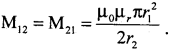Question 19.
Give the expression for maximum induced emf in an ac generator.
Induced emf e = em sin ωt where em is maximum induced emf = BANω.

Question 20.
Mention the phycial quantity in electromagnetism which is an analogue of mass in mechanics.
Self inductance plays the role of inertia, which is the electromagnetic analogue of mass in mechanics.

### 2nd PUC Physics Electro Magnetic Induction Two Marks Questions and Answers

Question 1.
State and explain Lenz’s law. (March 2014)
The direction of the induced current in a circuit is given by Lenz’s law. It states that the induced current is always in such a direction as to oppose the cause which is producing it. Lenz’s law is just an illustration of the law of conservation of energy. If dΦ is positive, then induced emf will be negative and if dΦ is negative then the induced emf will be positive.Question 2.
Draw a neat labelled diagram of an ac generator or dynamo.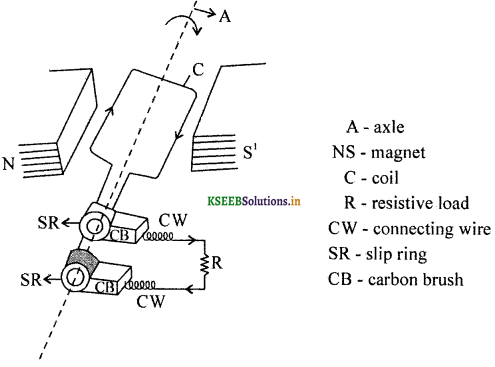Question 3.
Give the expression for energy required for the maximum Current in an inductor, or Write the expression for maximum energy stored in an inductor. (March 2014)
Energy required U = $$\frac { 1 }{ 2 }$$ LI2.
where, L – Self inductance of the coil and
I – maximum current.

Question 4.
Differentiate the terms self inductance and inductor.
Inductor is a device or a material which offers an opposition to the change in magnetic flux associated with it.
Self inductance is an intrinsic property of an inductor by virtue of which it opposes any change in magnetic flux in the inductor.

Question 5.
Say whether self inductance is a vector quantity or not. Write the dimensional formula for self inductance.
Ans:
Self inductance is a scalar physical quantity. Self inductance has the dimensions of [M1L2T-2A-2]Question 6.
Give the expression for the magnetic flux in terms of magnetic flux density and area.ΦB = $$\vec{B}$$.$$\vec{A}$$ = BA cos θ
Also ΦB = $$\Sigma \overrightarrow{\mathrm{B}}_{\mathrm{i}} . \mathrm{d} \overrightarrow{\mathrm{A}}_{\mathrm{i}}$$
where, Σ stands for summation over all the area elements

Question 7.
Express the unit ‘gauss’ (G) in terms of ‘tesla’ (T).
1 gauss = 10-4 tesla
i.e, 1G = 10-4 T.
e.g.: Horizontal component of earth’s magnetic field.
Bh = 0.38 × 10-4 T = 0.38 G.

Question 8.
Give the expression for joule heat in a rectangular conductor of length ‘l’ and resistance V moved at a speed of ‘v’ at right angle to the magnetic field ‘B’.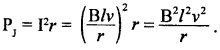Question 9.
Mention the expression for self inductance of a solenoid. Give the meaning of the symbols used.Where, N – Number of turns
A – Area of cross section .
l – length of a solenoid

Question 10.
If I1 and I2 represent currents in a pair of coils, then give the expression for the induced emf in any one coil.
Induced emf in the coil (1) is given by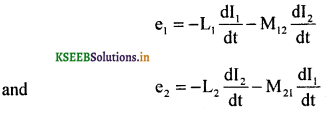Question 11.
What is the magnetic flux associated with the coil embedded in a constant magnetic field? Give the meaning of the symbols used.
Magnetic flux ΦB = BAN cos ωt where ‘N’ represents number of turns, B – magnetic flux density, A- area of the coil and ωt phase angle between the normal to the plane of the coil and the magnetic flux density pointing north pole to south pole.### 2nd PUC Physics Electro Magnetic Induction Three Marks Questions and Answers

Question 1.
What is electromagnetic induction? Explain.
The phenomenon in which an ernf and hence a current is induced in a closed circuit, due to a change in the magnetic flux linked with it, is called electromagnetic induction.

Whenever there is a relative motion between the magnet and the coil, a momentary current is induced, resulting in a momentary deflection in the galvanotneter. When the magnet moves towards the coil the magnetic flux linked with the coil increases, and when moved away from the coil, the flux decreases.

Question 2.
Give the expression for mutual inductance between a the pair of coaxial solenoids of same length. Give the meaning of the symbols used.
M12 M21 = $$\frac{\mu_{0} \mu_{\mathrm{r}} \mathrm{N}_{1} \mathrm{N}_{2} \mathrm{A}_{1}}{l}$$
Where, N1, N2 represent number of turns in coil (1) and (2) and A1 represents area of cross section of the inner solenoid.

Question 3.
Define self inductance of a solenoid. On what factors does it depend?
The phenomenon in which an emf is induced in a coil due to the change of current through it under resonance, is known as self induction. The property of a coil to oppose any change of current in it, is known as self inductance. The self inductance depends on,

• its length
• number of turns
• area of cross section and
• permeability of the material used as its core.

L = $$\frac{\mu_{0} \mu_{r} N^{2} A}{l}$$Question 4.
Define mutual inductance between a pair of coils. On what factors does it depend?
The phenomenon in which a change of current in one coil induces an emf in a neighbouring coil is called mutual induction. Mutual inductance between a pair of coils is numerically equal to the emf induced in the secondary coil when the current through the primary coil changes at unit rate.
Mutual inductance depends on,

• the number of turns in both the coils
• the area of the inner coil,
• the length of primary coil and
• the magnetic permeability of the core on which the primary is wound.

M = $$\frac{\mu_{0} \mu_{r} N_{1} N_{2} A}{l}$$

Question 5.
If a spoke is connected between the rim of the wheel and the centre (axle) and BH is the earth’s horizontal component of total field- perpendicular to the plane of the wheel and the wheel is rotated with an angular speed, then what will be the induced emf between the ends of the spoke?
Induced emf = $$\frac { 1 }{ 2 }$$BωR2.
Note induced emf ‘e’ = B $$\frac{d}{d t}$$ (area of a sector swept by the spoke between the two points on the rim)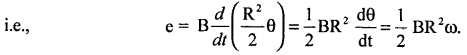Question 6.
What are eddy currents? Mention any two applications of eddy currents. (March 2014, July 2014)
The circulating currents set up in bulk pieces of metal through a magnetic field are called eddy currents.
Practical applications of eddy currents:
1. Magnetic brakes work on the principle of eddy currents. When a magnetic field is applied on the rotating drum, eddy currents are induced in the drum. The eddy currents decrease steadily in magnitude as the train slows down. Thus the braking effect is quite smooth.

2. Energy meters are instruments used to measure the electrical energy consumed by the electrical appliances. A metal disc in the energy meter rotates due to eddy currents. Other applications:

3. Induction (ac) motor (rotor) is a low resistance coil placed in a rotating magnetic field. Eddy currents in a rotor, rotates it in a direction of the magnetic field.

4. Eddy currents of high frequencies are used in the induction furnace which is used to, prepare alloys by melting the constituent metals.### 2nd PUC Physics Electro Magnetic Induction Five Marks Questions and Answers

Question 1.
Describe experiments to demonstrate electromagnetic induction.(a) Magnet and coil experiment: If one end of a magnet is suddenly introduced into a coil connected to a sensitive galvanometer it shows a momentary deflection.

This shows that a momentary current and hence an instantaneous emf is produced in the circuit and the direction of the current depends on the pole towards the coil. The current produced is called induced current. If the magnet is withdrawn suddenly, a sudden deflection is again observed but in the opposite direction. The deflection is greater if the magnet is introduced or withdrawn quickly. No induced emf is obtained when there is no relative motion between the coil and the magnet.

(b) Coil and coil experiment: Consider two coils P and S placed closed to each other. Coil P connected to a cell through a key Sw is called the primary coil. The other coil S connected in series with a sensitive galvanometer G is called the secondary coil. On pressing Sw a momentary deflection is noticed in the galvanometer. When the key is released the galvanometer deflects in the opposite direction. However, no induced emf is obtained when a steady current flows through the primary, as long as the secondary coil is at rest.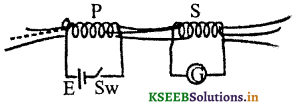Question 2.
What is meant by alternating current? Derive the expression for a sinusoidal e.m.f. induced in a coil rotating with uniform angular speed in a uniform magnetic field.
Current that changes in its direction once in every half cycle is called as alternating current. Let Magnetic flux, Φ = BAN cos cot ωt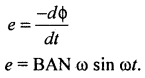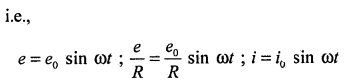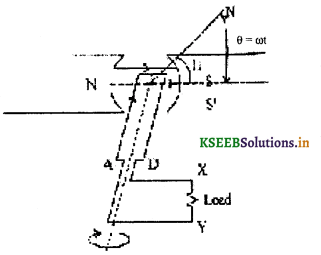The direction of ‘e’ is +ve for Φ = 0° to 180° and the induced current flows from X to Y and for Φ = 180° to 360°, the induced current flows from Y to X.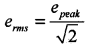Where, epeak = e0 = BAN ω
B – Magñetic flux density,
A – Area of the conductor,
N – No. of turns.
ω – Angular frequency of rotation.Question 3.
Obtain an expression for the motional electromotive force between the ends of a straight conductor moving in an uniform magnetic field at right angles to the field. (July 2014, March 2015)
Let ‘PQ’ represent a conductor moving with a speed ‘y’ at right angles to the magnetic flux density ‘B’ pointing perpendicular tõ the sheet of paper ami pointing towards the paper. Let RQ = x be the distance covered by the conducor. Magnetic flux ΦB = Blx.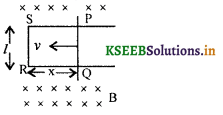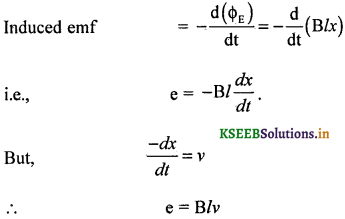Note : The Lorentz force acts on a charge in a conductor and acts along PQ. Work done in moving a charge ‘q’ from P to Q is
W = (Lorentz force) (displacement of the charge ‘q’)
i.e., W = (Bqv) lEquation (1) and (2) are identical.

Question 4.
Obtain an expression for energy stored in an inductor connected to a source voltage.
When a current grows through an inductor, the induced emf opposes its growth and as such it has to do some work. Let the current flowing through the circuit at any instant t be i.
The rate of growth of current at that time = $$\frac{d i}{d t}$$
The induced emf set up in the inductor E = $$L \frac{d i}{d t}$$
The work done by the current in increasing from zero to its maximum value I, W = $$\int_{0}^{1} d W$$This amount of work done is stored in the form of magnetic energy. We note that the self inductance is the electrical inertia which opposes the growth or decay of current in the circuit.### 2nd PUC Physics Electro Magnetic Induction List of Formulae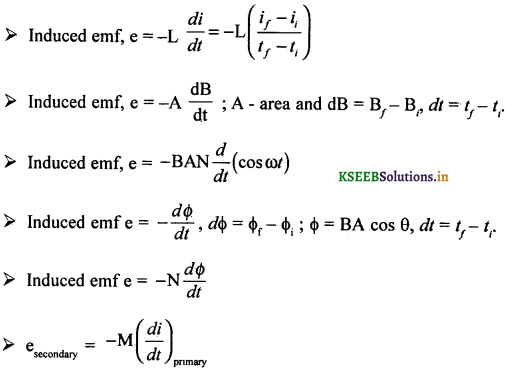### 2nd PUC Physics Electro Magnetic Induction Numericals and Solutions

Question 1.
Two coils are wound on the same iron rod so that the flux generated by one also passes through the other. The primary has 100 turns and the secondary has 200 turns. When a current of 2A flows through the primary, the flux in it is 2.5 × 10-4 Wb. Determine the value of mutual inductance (M) between the coils.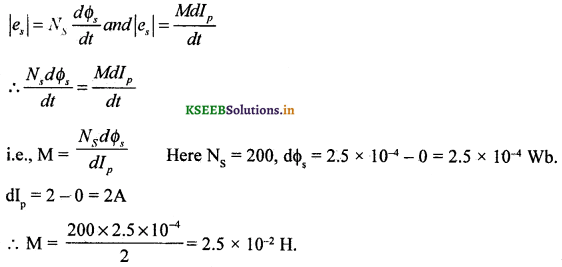Question 2.
A pair of adjacent coils have a mutual inductance of 0.25 II. If the current in the primary changes from zero to 2A in 0.05 sec., what is the average induced emf in the secondary?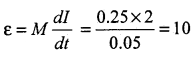Induced emf in the secondary = 10 V.

Question 3.
A solenoid 0.70m in length and of 2100 turns has a radius 4.5 × 10-2 m. A second coil of 750 turns is wound over the middle part of the solenoid. Find the self inductance of the solenoid and mutual inductance between the two coils.
Given, l = 0.70m. N1 = 2100, r = 4.5 × 10-2 m N2 = 750, A = πr2.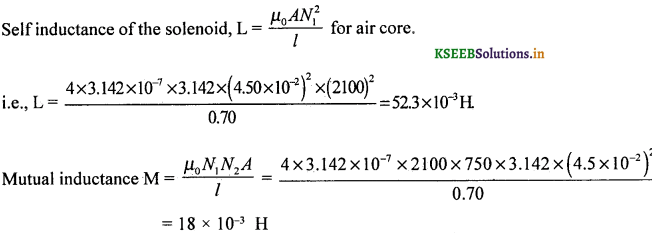Question 4.
A solenoid having a core of cross-section 4 × 10-4 m2, half air and half iron (µr = 500) is 0.22 m long. If the number of turns in it is 1000, then calculate the self inductance.Question 5.
The current in a coil of 20 mH changes from 2.5A to 3A in $$\frac{1}{100}$$ second. Find the induced emf.
Given: L = 2 0 mH = 2 × 10-2 H, dl = l2 – l1 = 3 – 2.5 = 0.5 A, dt = $$\frac{1}{100}$$ s = 10-2 s, e = ?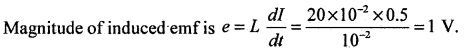Question 6.
Current in a coil changes from 4 A to 0 A in 0.1 s. If the average emf induced is 100 volts, what is the self inductance of the coil?Question 7.
(a) Calculate the self inductance of a solenoid of length 0.30 m containing 1000 turns and having area of cross section 36 cm2.
(b) Calculate the induced emf in the solenoid, if the current decreases at the rate of 100 A s-1.
(a) Given l = 0.3 m, N = 103 turns, A= 36 × 10-4 m2, $$\frac{d i}{d t}$$ = – 100A s-1(b) Induced emf e = -L$$\frac{d i}{d t}$$
= -1.508 × 10-2 (-100)
= +1.508 VQuestion 8.
A small dc motor operating at 200 V draws a current of 5.0 A at its full speed of 100 π rad s-1. The resistance of the armature of the motor is 10Ω. Determine the back emf of the motor. Obtain the power input, power output and efficiency of the motor.
Given : V = 200 V, I = 5.0 A, R = 10Ω, ω = 100π rad s-1.
If’ ‘ε’ is the back emf then,
V – ε = IR (from KVL)
∴ ε = V – IR = 200 – (5)(10) = 150 V.
Input power, VI = 200 × 5 = 1000 W
Output power, εi = 150 × 5 = 750W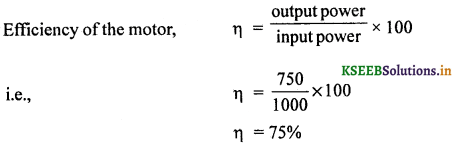Question 9.
Calculate the equivalent inductance between A and B from the following circuit.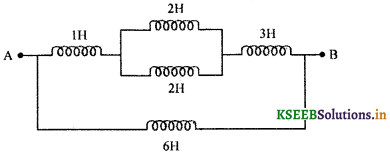Question 10.
A square metal wire loop of side 0.10 m and resistance 1Ω is moved at a constant velocity v0 in a uniform magnetic field of induction B = 2 Wb m-2 as shown in the figure. The magnetic field lines are ⊥r to the plane of the loop directed into the paper. The loop is connected to a network of resistors each of value 3Ω. The resistance of lead wires OS and PQ are negligible. What should be the speed v0 of the loop so as to have a steady current of 1mA in the loop? Also indicate the direction of current in the loop.The equivalent resistance of the network = 3Ω
The resistance of the loop = 1 Ω
Total resistance R = 3 + 1 = 4Ω
Electro motive force in the loop = e = Blv0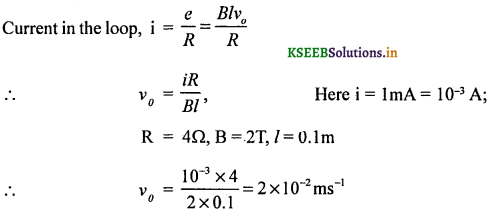Question 11.
A long solenoid with 15 turns per cm has a small loop of area 2.0 cm2 placed inside the solenoid normal to its axis. If the current carried by the solenoid changes steadily from 2.0 A to 4.0 A in 0.1s, then what is the induced emf in the loop while the current is changing?
GivenQuestion 12.
A rectangular wire loop of sides 8 cm and 2 cm with a small cut is moving out of region of uniform magnetic field of magnitude 0.3T directed normal to the loop. What is the voltage developed across the cut if the velocity of the loop is 1 cm s-1 in a direction normal to the :
(i) longer side
(ii) shorter side of the loop? For how lone does the induced voltage last in each case?
Given : l = 8 × 10-2 m, b = 8 × 10-2m,
B = 0.3 T, v = 10-2 ms-1
Let ‘e’ be induced emf developed across the cut in the loop.

(i) e1 = Blv = 0.3 × 8 × 10-2 × 10-2V = 2.4 × 10-4 V
The induced emf will last for the time during which the breath of the loop moves out of the field.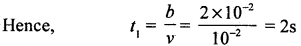(ii) e2 = B/v = 0.3 × 2 × 1o-2 × 10-2 V
= 6.0 × 10-5 V.
The induced emf will last for the time during which the length of the loop moves out of the field.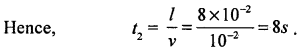Question 13.
A circular coil of radius 8.0. cm and 20 turns, is rotated about its vertical diameter with an angular speed of 50 rad s-1 in a uniform horizontal magnetic field of magnitude 3.0 × 10-2 T. Obtain the maximum and average emf induced in the coil. If the coil forms a closed loop df resistance 10Ω, calculate the maximum value of current in the coil. Calculate the average power loss due to Joule heating. Where does this power come from?
Given : N = 20, R = 8 × 10-2 m, ω = 50 rad s-1,
B = 3 × 10-2T, resistance r = 10Ω
imax = ? $$\bar{p}$$ = ?
Magnetic flux, Φ = BAN cos ωt ; A = πR2
= 3 × 10-2 × 3.14 × 64 × 10-2 × 20 cos ωt
Φ = 0.0120ó cos ωt
Φ = $$-\frac{d \phi}{d t}$$ = -0.01206ω sin ωt
e = -0.01206 x 50 sin ωt
e = -0.603 sin ωt
∴ em = 0.603VAverage emf over a complete cycle is zero.This power comes from the external rotor.

Question 14.
A horizontal straight wire 10 m long extending from east to west is falling with a speed of 5.0ms1 at right angles to the horizontal component öf the Earth’s magnetic field 0.30 × 10-4 Wb m-2.
(a) What is the instantaneous value of the emf induced in the wire?
(b) What is the direction of the emf?
(c) Which end of the wire is at the higher electrical potential?
Given: B 0.3 × 10-4 Wbm-4, l = 10m
v = 5 ms, θ = 90°
(a) w.k.t e = B/v sin θ
i.e., e = 0.3 × 10-4 × 10 × 5 × 1
= 1.5 × 10-3V
(b) The direction of emf is from west to east .
(c) The east end will be at a higher electrical potential (-ve potential). The conventional current flows from +ve(East) end to -ve(West) end of the wire.Question 15.
An air cored solenoid with length 30 cm, area of cross section 25cm2 and number of turns 500 carries a current of 2.5 A. The current is suddenly switched off in a brief time of 10-3. What is the average back emf induced across the ends of the open switch in the circuit?
Magnetic field in a solenoid B = $$\frac{\mu_{0} \mathrm{N} i}{l}$$